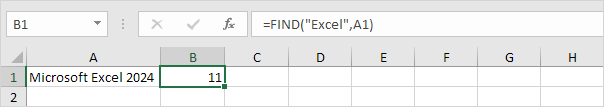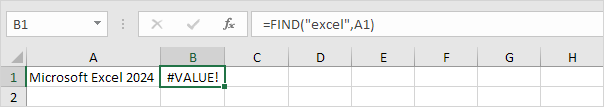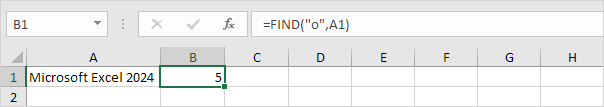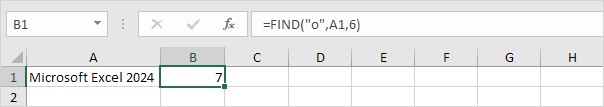# Find

Use the FIND function in Excel to find the position of a substring in a string. The FIND function is case-sensitive.

1. The FIND function below finds the string "ro" at position 4.2. The FIND function below finds the string "Excel" at position 11.3. The FIND function is case-sensitive. The FIND function below returns the #VALUE! error because it cannot find the string "excel".The FIND function has a 3rd optional argument. You can use this argument to indicate the position, counting from the left, at which you want to start searching.

4. The FIND function below finds the string "o" at position 5.5. The FIND function below starts searching at position 6 and finds the string "o" at position 7.Note: the SEARCH function in Excel is case-insensitive and supports wildcards.

Go to Next Chapter: Lookup & Reference Functions   |   Become an Excel Pro: 300 Examples